# How to use the Excel OFFSET functionThe Excel OFFSET function returns a reference range of cells that are offset (hence the name) from a reference cell. In other words, you specify the offset in terms of the number of rows and columns, and you can also indicate the size of the array that you want to reference.

### Syntax

=OFFSET(reference, rows, cols, [height], [width])
• reference is the starting cell from which the results are offset
• rows is the number of rows you want to offset from the reference cell
• cols is the number of columns you want to offset from the reference cell
• height is the height of the array you want to return. The default is 1
• width is the width of the array you want to return. The default is 1

### How to use the Excel OFFSET function

Now I’ll show the function in action. Take a look at the example below.

I have some data from a clothing store that indicates how many of each item has been sold, per size. I use the OFFSET function to reference data within this table.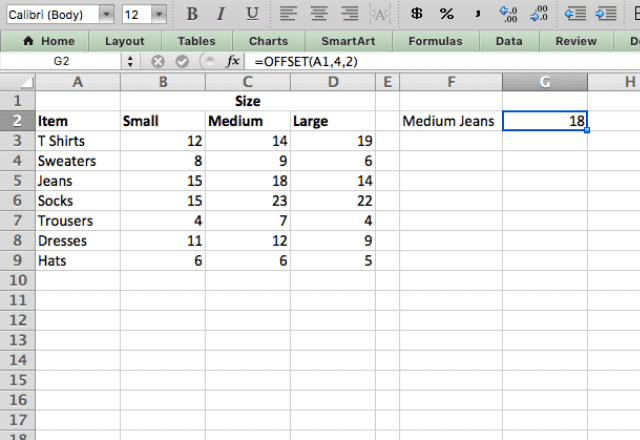As you can see, I type the function =OFFSET(A1,4,2) to pull the data I want from the table. In this case, I start with a reference cell of A1, then tell the function to move 4 rows down and 2 rows across. As a result, it performs that offset and returns the results from cell C5.

If I want to get the sales of large T-shirts, I can use the formula =OFFSET(A1,2,3). This function is the same as in the previous case, except I tell the function to offset 2 rows down and 3 columns to the right of cell A1.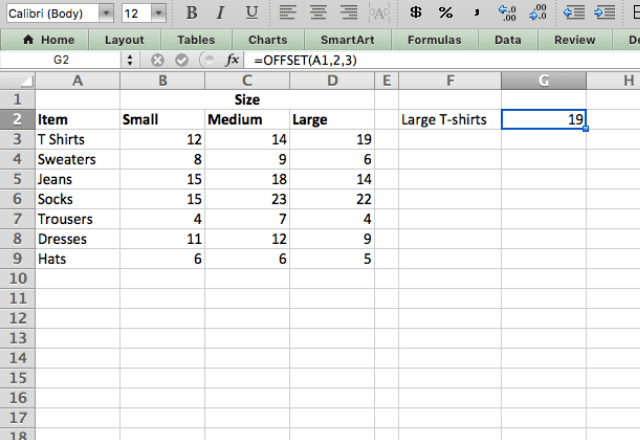Notice how I didn’t input the two optional parameters, [height] and [width]. This is because I only want to return 1 cell, so I don’t have to input anything for these since they default to 1.

If I wanted to return multiple cells however, I would specify the dimensions of the desired output.

#### Using OFFSET with other functions

The OFFSET Excel function can also be combined with other functions.

In this example, I combine OFFSET with the SUM function to get the total sales of Large clothing.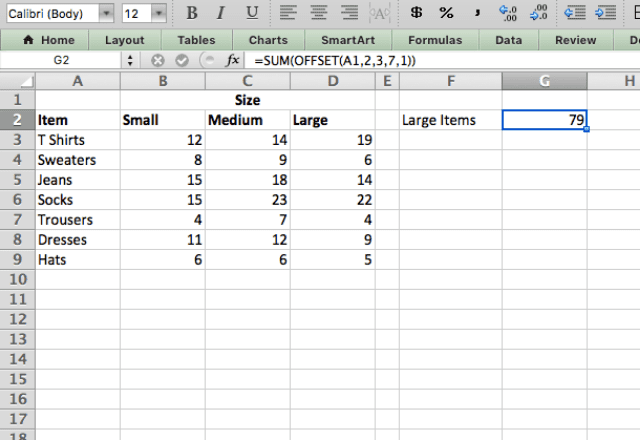The formula here is =SUM(OFFSET(A1,2,3,7,1)). This time, I input 7 for the height parameter. This makes the function return the range D3:D9, which encompasses all the large items. It then adds them all up with the SUM function.

If you’re still confused about this example, here’s what we tell the function told to do: start at cell A1, then move 2 rows down, 3 rows across, and return the results from an array of 7 (height) by 1 (width).

Finally, the SUM function then adds up this array (D3:D9).

#### Using OFFSET to create a dynamic range

One of the main uses of the OFFSET function is to create a dynamic range. A dynamic range is a range that updates automatically when new data is added.

Take a look at the example below. Here, I use the OFFSET function to create a dynamic range of the entire table that will update if new data is inserted.

Lastly, I combine this with the AVG function to get the average sales per item.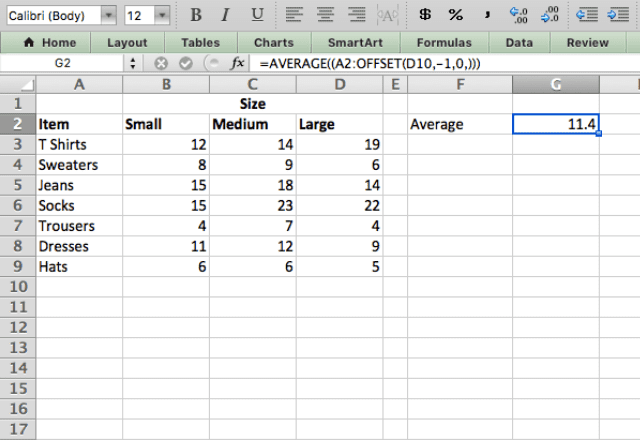This is achieved with the formula =AVERAGE((A2:OFFSET(D10,-1,0,))). I use the OFFSET function to identify the lower bound of the range I want to average.

The range goes from cell A2 (fixed) to one cell above D10. The benefit here is that if I insert a new row, the function will automatically update: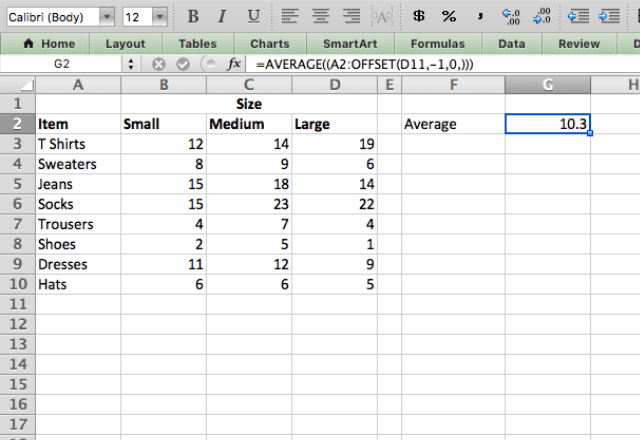The formula here is =AVERAGE((A2:OFFSET(D11,-1,0,))). The OFFSET updated from D10 to D11, which kept the entire table included in the average calculation. I didn’t change the formula – I just added a new row (shoes) and the function updated automatically!

I hope you now have an understanding of how and when to use the OFFSET function!

### OFFSET function

And there you go! Use the OFFSET function to return a reference range of cells that are offset from a reference cell.

If you’d like to learn more about the various formulas of Google Sheets, why not take a look at our blog post on the ARRAY_CONSTRAIN formula in Google Sheets.

Alternatively, check out related blog posts below!